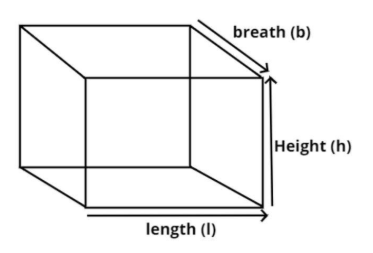QuestionAnswers

# Find the L.S.A (lateral surface area) of a cuboid whose dimensions are given by $3m \times 5m \times 4m$.Verified
113.4K+ Views
Hint – In this question use the direct formula of lateral surface area (L.S.A) of a cuboid which is $2\left( {lh + bh} \right)$. The given sides are of the form $l \times b \times h$.The pictorial representation of the cuboid is shown above.
As we know that in the lateral surface area top and bottom areas are excluded.
So the required lateral surface area of the cuboid has four faces.
So the lateral surface area (L.S.A) of the cuboid is = $2\left( {lh + bh} \right)$ sq. units.
Now it is given that the dimension of cuboid are $\left( {3m \times 5m \times 4m} \right)$
So the length (l) of the cuboid = 3 meter.
Breadth (b) of the cuboid = 5 meter.
And height (h) of the cuboid = 4 meter.
So the lateral surface area of the cuboid is
$\Rightarrow L.S.A = 2\left[ {\left( {3 \times 4} \right) + \left( {5 \times 4} \right)} \right]$
Now simplify the above equation we have,
$\Rightarrow L.S.A = 2\left[ {12 + 20} \right] = 2\left( {32} \right) = 64{\text{ }}{{\text{m}}^2}$
So the required lateral surface area of the cuboid is 64 square meter.
So this is the required answer.

Note – Lateral surface area is finding the area of all the sides of the object excluding its base and top (when they exist) whereas the total surface area of any solid is the sum of areas of all faces of the solid including the base and the top.